## If (3x − 2)(3x + 2) = ax2 − b, what is the value of a?

Question

If (3x − 2)(3x + 2) = ax2 − b, what is the value of a?

in progress 0
2 months 2021-07-24T14:54:03+00:00 2 Answers 3 views 0

1. (3x-2)(3x+ 2)

Multiply each term in one set of parentheses by the other terms:

3x x 3x =9x^2

3x x 2 = 6x

-2 x 3x = -6x

-2 x 2 = -4

Combine to get:

9x^2 + 6x – 6x -4

Combine like terms:

9x^2 – 4

The value of a would be 9.

2.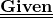• (3x − 2)(3x + 2) = ax2 − b⠀⠀⠀⠀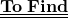• ⠀what is the value of a?⠀⠀⠀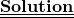At first we have to solve the value of (3x-2)(3x+2)

•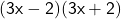•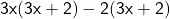•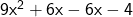•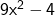According to the question,

•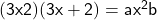•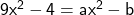•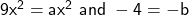•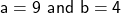⠀⠀⠀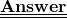Hence,

The value of a is 9